Warning: Illegal string offset 'html' in /home/hsn/public_html/forum/cache/skin_cache/cacheid_1/skin_topic.php on line 909

Algebra/Factorising Question (or at least I think it is :s) - HSN forum# Algebra/Factorising Question (or at least I think it is :s)

8 replies to this topic

• Location:UK
• Gender:Not Telling

Posted 15 March 2006 - 08:18 PM

Hey, can somebody complete & explain how this question is done? I'd be very greatful, cheers:(Area of the first rectangle is A1 and area of the second is A2)

A1 - A2 = x2 - (8p + 4)x - 8p

Given that A1-A2 = 1cm2, establish the value of p, where p>-1, for this equation to have only one solution for x.

And hence find x when p takes this value.

### #2dfx

Fully Fledged Genius

•• Members
•• 1,955 posts
• Gender:Male

Posted 15 March 2006 - 10:15 PM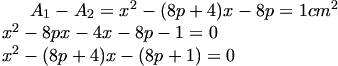This equation will only have one solution (of x) if the discriminant = 0 i.e. real and equal roots (effectively one root).

So solve for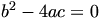and get your value of p.

And then just plug it back in for the value of x and solve the original quadratic.

• Location:UK
• Gender:Not Telling

Posted 16 March 2006 - 06:46 PMThis equation will only have one solution (of x) if the discriminant = 0 i.e. real and equal roots (effectively one root).

So solve forand get your value of p.

And then just plug it back in for the value of x and solve the original quadratic.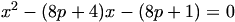How did you manage to arrive at this?Edit: Bleh, not sure if I'm doing this right...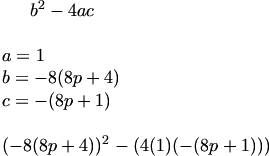Correct so far?

### #4Dave

Ruler (but not owner) of hsn

•• Moderators
•• 4,252 posts
• Location:kilmarnock(ok kilmaurs)
• Interests:programming, exercising, brass band, using this board
• Gender:Male

Posted 16 March 2006 - 07:31 PM

the line you dont understand comes about because there is a common factor of X in there. This makes sense as a quadratic is in the form Ax2+bx + c. noteing that p is just a number so -8p-1 is a constant

and b = -(8p+4)

If i am not here i am somewhere else

• Location:UK
• Gender:Not Telling

Posted 16 March 2006 - 08:06 PM

the line you dont understand comes about because there is a common factor of X in there. This makes sense as a quadratic is in the form Ax2+bx + c. noteing that p is just a number so -8p-1 is a constant

and b = -(8p+4)

Ah, yeah, silly me. I wrote it down correct when I was doing the calculation but typed it out wrong.Anyhoo, I got: p = -20/8 and p = -4 for some reason.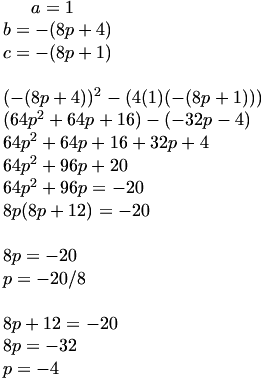Er, what did I do wrong this time?

Top of the Class

•• Members
•• 390 posts
• Location:Cambridge
• Interests:Muzak.
• Gender:Male

Posted 16 March 2006 - 09:18 PM

the line you dont understand comes about because there is a common factor of X in there. This makes sense as a quadratic is in the form Ax2+bx + c. noteing that p is just a number so -8p-1 is a constant

and b = -(8p+4)

Ah, yeah, silly me. I wrote it down correct when I was doing the calculation but typed it out wrong.Anyhoo, I got: p = -20/8 and p = -4 for some reason.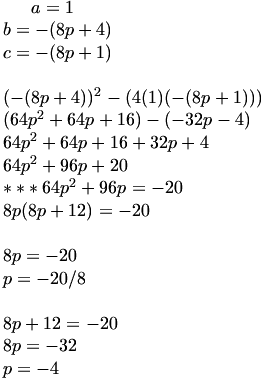Er, what did I do wrong this time?

I put *** where I think you went wrong - well this line is still correct but it the line following it is wrong. Keep the 64p^2 + 96p + 20 equal to zero, take out a common factor of four to get 4(16p^2 + 96p + 20) = 0. Now you have to split the part inside the bracket into two different brackets, and then find the values for p which make the brackets, and therefore the whole thing, equal to zero. One of them should be greater than -1.
HMFC - Founded 1874, beefing the Cabbage since 1875

• Location:UK
• Gender:Not Telling

Posted 16 March 2006 - 11:51 PM

the line you dont understand comes about because there is a common factor of X in there. This makes sense as a quadratic is in the form Ax2+bx + c. noteing that p is just a number so -8p-1 is a constant

and b = -(8p+4)

Ah, yeah, silly me. I wrote it down correct when I was doing the calculation but typed it out wrong.Anyhoo, I got: p = -20/8 and p = -4 for some reason.Er, what did I do wrong this time?

I put *** where I think you went wrong - well this line is still correct but it the line following it is wrong. Keep the 64p^2 + 96p + 20 equal to zero, take out a common factor of four to get 4(16p^2 + 96p + 20) = 0. Now you have to split the part inside the bracket into two different brackets, and then find the values for p which make the brackets, and therefore the whole thing, equal to zero. One of them should be greater than -1.

I think you're right - I tried that and got an answer of -0.25, so erm, yea. Thanks everybody.### #8John

HSN Legend

•• Members
•• 2,713 posts
• Location:In a Peripheral Estate in Glasgow
• Interests:First the boring stuff:<br /><br />2004 Results:<br /><br />Highers:<br /><br />Computing A<br />Craft and Design A<br />Physics A<br />Administration A<br />English A<br />Maths A<br />Modern Studies A<br />French A<br /><br />2005 Results:<br /><br />Advanced Highers:<br />Computing A<br />Physics A<br />Maths A<br />Modern Studies A<br />English A<br /><br />2006 Results:<br /><br />Advanced Highers(All Crashed):<br />Chemistry A<br />Biology A<br />Business Managemnt A<br /><br />Aren't I marvellous?
• Gender:Male

Posted 17 March 2006 - 01:14 PM

the line you dont understand comes about because there is a common factor of X in there. This makes sense as a quadratic is in the form Ax2+bx + c. noteing that p is just a number so -8p-1 is a constant

and b = -(8p+4)

Ah, yeah, silly me. I wrote it down correct when I was doing the calculation but typed it out wrong.Anyhoo, I got: p = -20/8 and p = -4 for some reason.Er, what did I do wrong this time?

I put *** where I think you went wrong - well this line is still correct but it the line following it is wrong. Keep the 64p^2 + 96p + 20 equal to zero, take out a common factor of four to get 4(16p^2 + 96p + 20) = 0. Now you have to split the part inside the bracket into two different brackets, and then find the values for p which make the brackets, and therefore the whole thing, equal to zero. One of them should be greater than -1.

But if you take out a factor of 4 you get 4(16p^2 + 24p + 5)
BeboTop of the Class

•• Members
•• 390 posts
• Location:Cambridge
• Interests:Muzak.
• Gender:Male

Posted 18 March 2006 - 10:39 AM

the line you dont understand comes about because there is a common factor of X in there. This makes sense as a quadratic is in the form Ax2+bx + c. noteing that p is just a number so -8p-1 is a constant

and b = -(8p+4)

Ah, yeah, silly me. I wrote it down correct when I was doing the calculation but typed it out wrong.Anyhoo, I got: p = -20/8 and p = -4 for some reason.Er, what did I do wrong this time?

I put *** where I think you went wrong - well this line is still correct but it the line following it is wrong. Keep the 64p^2 + 96p + 20 equal to zero, take out a common factor of four to get 4(16p^2 + 96p + 20) = 0. Now you have to split the part inside the bracket into two different brackets, and then find the values for p which make the brackets, and therefore the whole thing, equal to zero. One of them should be greater than -1.

But if you take out a factor of 4 you get 4(16p^2 + 24p + 5)Oops, I can never put down what I mean when I'm typing maths. Thanks for pointing that out.

HMFC - Founded 1874, beefing the Cabbage since 1875

#### 1 user(s) are reading this topic

0 members, 1 guests, 0 anonymous users